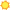# Mathematicians15 matches for financial math

 O*NET-SOC Title 1 match Alternate Occupation Titles 5 matches O*NET-SOC Description 1 match Tasks 6 matches Detailed Work Activities 2 matches

## O*NET-SOC Title

• Mathematicians

## Alternate Occupation Titles

• Mathematician
• Applied Mathematician
• Associate Professor of Finance
• Computational Mathematician
• Engineering Mathematician

## O*NET-SOC Description

• Conduct research in fundamental mathematics or in application of mathematical techniques to science, management, and other fields. Solve problems in various fields using mathematical methods.

• Apply mathematical theories and techniques to the solution of practical problems in business, engineering, the sciences, or other fields.
• Conduct research to extend mathematical knowledge in traditional areas, such as algebra, geometry, probability, and logic.
• Design, analyze, and decipher encryption systems designed to transmit military, political, financial, or law-enforcement-related information in code.
• Develop mathematical or statistical models of phenomena to be used for analysis or for computational simulation.
• Develop new principles and new relationships between existing mathematical principles to advance mathematical science.
• Maintain knowledge in the field by reading professional journals, talking with other mathematicians, and attending professional conferences.

## Detailed Work Activities

• Apply mathematical principles or statistical approaches to solve problems in scientific or applied fields.
• Develop scientific or mathematical models.